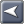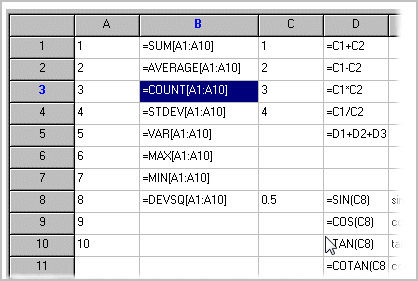Demographic Data Analyst»No topics above this level«SpreadGrids are used in many DDA components.  This section provides an overview of features and operations.

simple formula editing interface

auto recalculation

native XLS file import and export

single cell recalculation, full recalculation

extensive range of mathematical functions

save with formulas or formula results only

single cell references in formulas

cell range formulas

formula precision for grid on cell basis

display formulas or formula results

date / time functions

intelligent formula aware copy and paste

can be extended with custom functions

cell names

cell name mode can be RxCy style or A1-style

can reference cells from other SpreadGrids

math library infrastructure to allow easy extending